# JAVA之旅（二）——if,switch,for，while,do while,语句嵌套，流程控制break , continue ，函数，重载的示例总结JAVA之旅（二）——if,switch,for，while,do while,语句嵌套，流程控制break , continue ，函数，重载的示例总结http://www.bieryun.com/2615.htmlJAVA的思想真的很重要，所以要专心的学——献给刚入门的小程序员们

## 一.语句

• 判断语句 if
• 选择语句 switch
• 循环语句 for
• 当然，还有其他的

### 1.if

if,如果，就是判断，if(条件){}

``````//公共的   类   类名
public class HelloJJAVA {
// 公共的 静态 无返回值 main方法 数组
public static void main(String[] str) {
int a = 5;
if (a > 10) {
System.out.println("我比10大");
} else {
System.out.println("我比10小");
}
}
}``````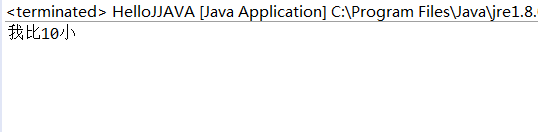``````//公共的   类   类名
public class HelloJJAVA {
// 公共的 静态 无返回值 main方法 数组
public static void main(String[] str) {
// 根据1-7来判断使星期几

int day = 3;

// 判断
if (day == 1) {
System.out.println("今天星期一");
} else if (day == 2) {
System.out.println("今天星期二");
} else if (day == 3) {
System.out.println("今天星期三");
} else if (day == 4) {
System.out.println("今天星期四");
} else if (day == 5) {
System.out.println("今天星期五");
} else if (day == 6) {
System.out.println("今天星期六");
} else if (day == 7) {
System.out.println("今天星期七");
} else {
System.out.println("不是1-7范围内的数字");
}

}
}``````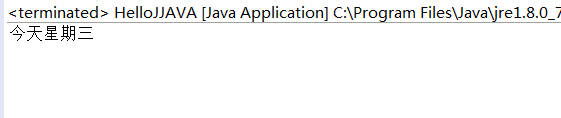### 2.switch

``````switch (表达式) {
case 取值1:

break;
//最终执行
default:
break;
}``````

``````//公共的   类   类名
public class HelloJJAVA {
// 公共的 静态 无返回值 main方法 数组
public static void main(String[] str) {

int a = 3;

switch (a) {
case 1:
System.out.println("1");
break;
case 2:
System.out.println("2");
break;
case 3:
System.out.println("3");
break;
case 4:
System.out.println("4");
break;
case 5:
System.out.println("5");
break;
// 最终执行
default:
System.out.println("都不是");
break;
}

}
}``````

``````//公共的   类   类名
public class HelloJJAVA {
// 公共的 静态 无返回值 main方法 数组
public static void main(String[] str) {

/**
* 根据用户指定的月份，打印相应的季节
*/

int month = 7;

switch (month) {
case 1:
case 2:
case 3:
System.out.println("春");
break;
case 4:
case 5:
case 6:
System.out.println("夏");
break;
case 7:
case 8:
case 9:
System.out.println("秋");
break;
case 10:
case 11:
case 12:
System.out.println("冬");
break;

default:
System.out.println("输入不再范围内");
break;
}

}
}``````

if和switch很像，那什么时候使用呢？如果判断的具体数值不多，而是符合byte short int char 类型，使用switch，其他情况，使用if，虽然都可以，但是switch效率据说稍微高一点点…

### 3.while

• while
• do while
• for

``````while (条件) {
// 输出

}``````

``````//公共的   类   类名
public class HelloJJAVA {
// 公共的 静态 无返回值 main方法 数组
public static void main(String[] str) {

int a = 5;
// 循环5次
while (a < 10) {
a++;
System.out.println("a = " + a);
}

}
}``````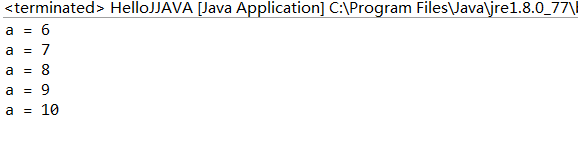### 4. do while

do while要结合while语句这样更容易说明一些事情

``````//公共的   类   类名
public class HelloJJAVA {
// 公共的 静态 无返回值 main方法 数组
public static void main(String[] str) {

int a = 1;
do {
System.out.println("a = " + a);
a++;
} while (a < 10);
}
}``````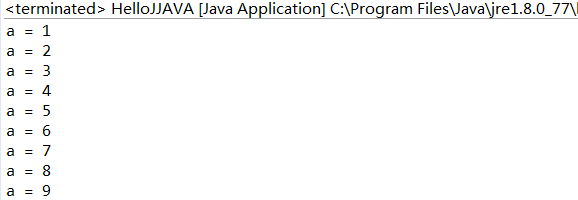### 5.for

``````
/**
* 条件表达式 循环条件表达式  循环后的操作表达式
*/
for (int i = 0; i < str.length; i++) {
//执行语句
}``````

``````//公共的   类   类名
public class HelloJJAVA {
// 公共的 静态 无返回值 main方法 数组
public static void main(String[] str) {

/**
* 条件表达式 循环条件表达式 循环后的操作表达式
*/
for (int i = 0; i < 10; i++) {
// 执行语句
System.out.println("i = " + i);
}
}
}``````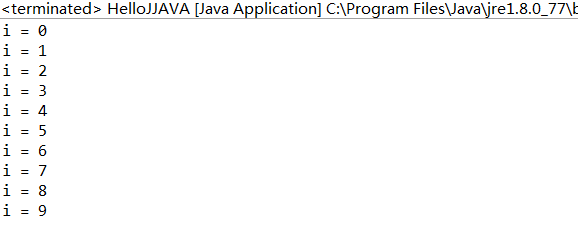``````//公共的   类   类名
public class HelloJJAVA {
// 公共的 静态 无返回值 main方法 数组
public static void main(String[] str) {

int i = 0;
while (i < 10) {
System.out.println("i = " + i);
i++;
}
}
}``````

• 作用域不同

``````//公共的   类   类名
public class HelloJJAVA {
// 公共的 静态 无返回值 main方法 数组
public static void main(String[] str) {

/**
* 获取1-10的和并且打印
*/
// 用于存储不断变化的和
int sum = 0;
// 记录累加的值
for (int i = 1; i < 11; i++) {
sum += i;
}
System.out.println("和为："+sum);
}
}``````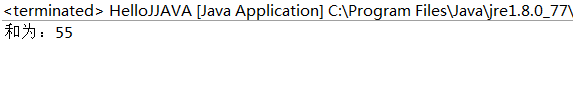``````//公共的   类   类名
public class HelloJJAVA {
// 公共的 静态 无返回值 main方法 数组
public static void main(String[] str) {
/**
* 打印1-100之间7倍数的个数
*/

int temp = 0;
for (int i = 1; i <= 100; i++) {
if (i % 7 == 0) {
temp++;
}
}
System.out.println("个数为：" + temp);
}
}``````

### 6.语句嵌套

``````//公共的   类   类名
public class HelloJJAVA {
// 公共的 静态 无返回值 main方法 数组
public static void main(String[] str) {
for (int i = 0; i < 3; i++) {
for (int j = 0; j < 4; j++) {
System.out.println("Hello");
}
}
}
}``````

``````//公共的   类   类名
public class HelloJJAVA {
// 公共的 静态 无返回值 main方法 数组
public static void main(String[] str) {
for (int i = 0; i < 4; i++) {
for (int j = 0; j < 4; j++) {
System.out.print("*");
}
//换行
System.out.println();
}
}
}````````````//公共的   类   类名
public class HelloJJAVA {
// 公共的 静态 无返回值 main方法 数组
public static void main(String[] str) {
for (int i = 0; i < 10; i++) {
for (int j = 0; j < i; j++) {
System.out.print("*");
}
// 换行
System.out.println();
}
}
}``````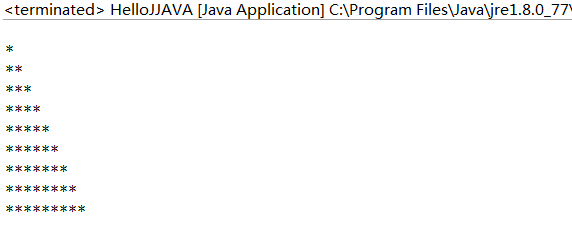``````//公共的   类   类名
public class HelloJJAVA {
// 公共的 静态 无返回值 main方法 数组
public static void main(String[] str) {
for (int i = 0; i < 10; i++) {
for (int j = i; j < 10; j++) {
System.out.print("*");
}
// 换行
System.out.println();
}
}
}``````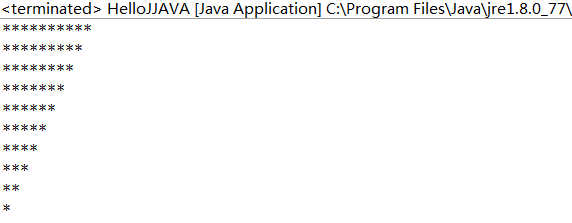``````//公共的   类   类名
public class HelloJJAVA {
// 公共的 静态 无返回值 main方法 数组
public static void main(String[] str) {
for (int i = 0; i < 6; i++) {
for (int j = 1; j < i; j++) {
System.out.print(" "+j);
}
System.out.println();
}
}
}``````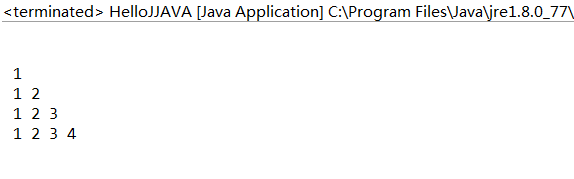``````import java.time.Year;

//公共的   类   类名
public class HelloJJAVA {
// 公共的 静态 无返回值 main方法 数组
public static void main(String[] str) {
/**
* 九九乘法表
*/
for (int i = 1; i < 10; i++) {
for (int j = 1; j < i + 1; j++) {

System.out.print(j + "*" + i + " = " + j * i + " ");
}
System.out.println(" ");
}
}
}``````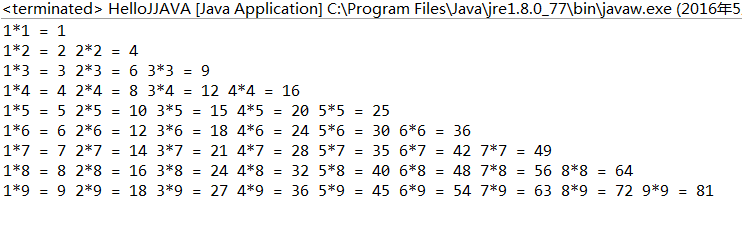### 7.流程控制语句

• break : 跳出 选择和循环结构
• continue: 继续 循环结构

``````//公共的   类   类名
public class HelloJJAVA {
// 公共的 静态 无返回值 main方法 数组
public static void main(String[] str) {
for (int i = 0; i < 3; i++) {
System.out.println("i : " + i);
// 跳出循环
break;
}
}
}``````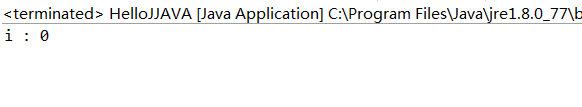``````//公共的   类   类名
public class HelloJJAVA {
// 公共的 静态 无返回值 main方法 数组
public static void main(String[] str) {
for (int i = 0; i < 10; i++) {

if (i % 2 == 1) {
// 继续循环
continue;
}
System.out.println("i : " + i);
}
}
}``````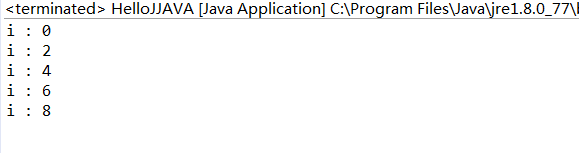``````//公共的   类   类名
public class HelloJJAVA {
// 公共的 静态 无返回值 main方法 数组
public static void main(String[] str) {
/**
* 等腰三角形
*/
for (int i = 0; i < 5; i++) {
for (int j = i + 1; j < 5; j++) {
System.out.print(" ");
}
for (int z = 0; z <= i; z++) {
System.out.print("* ");
}
System.out.println();
}
}
}``````

OK，结束## 二.函数

``````//公共的   类   类名
public class HelloJJAVA {
// 公共的 静态 无返回值 main方法 数组
public static void main(String[] str) {
/**
* 求任何数+5的和
*/
System.out.println(getNum(5));
}

private static int getNum(int a) {
return a + 5;
}
}``````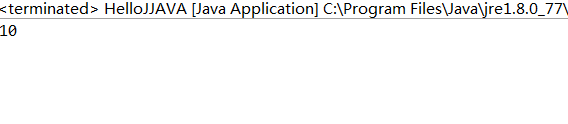• 便于对该功能进行复用
• 函数只有被调用的时候才会执行
• 函数的出现提高了代码的复用性
• 对于函数没有具体返回值的情况，返回值类型可以用void，那么return就可以不用写了

• 函数中只能调用函数，不可以在函数内部定义函数
• 定义函数时，函数的结果返回给调用者，交由调用者处理

``````//公共的   类   类名
public class HelloJJAVA {
// 公共的 静态 无返回值 main方法 数组
public static void main(String[] str) {

System.out.println(getNum());
}

private static int getNum() {
return 6 + 5;
}
}``````

## 三.重载

• 概念

在同一个类中，允许存在一个以上的同名函数，只要他们的参数或者参数类型不同即可

• 特点

与返回值类型无关，只看参数列表

• 好处
方便阅读，优化程序设计

``````//公共的   类   类名
public class HelloJJAVA {
// 公共的 静态 无返回值 main方法 数组
public static void main(String[] str) {

}

private static int getNum(int a) {
return a;
}

private static int getNum(int a, int b) {
return a + b;
}

private static int getNum(int a, int b, int c) {
return a + b + c;
}

}``````

OK，这个重载的示例就不写了，我们本篇就先到这里吧，下节我们讲数组之类的数据处理，嘻嘻！• 回顶